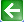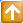Satish Lele lelepiping@gmail.com

Estimation of Insulation Thickness, Optimum Thickness
A standardized insulation rating system ensures consistency, with insulating materials rated in R- and U-values. The R-value is a measure of thermal resistance, represent resistance to the flow of heat. The higher the R-value, the greater the resistance and the insulating value. U-values are the direct opposite, representing the amount of heat that escapes through a material. The lower the U-value, the slower the rate of heat flow and the better the insulating quality.
It is expressed as the thickness of the material divided by the thermal conductivity. For the thermal resistance of an entire section of material, instead of the unit resistance, divide the unit thermal resistance by the area of the material. If you have the unit thermal resistance of a wall, divide it by the cross-sectional area of the depth of the wall to compute the thermal resistance. The unit thermal conductance of a material is denoted as C and is the reciprocal of the unit thermal resistance. This can also be called the unit surface conductance, commonly denoted by h.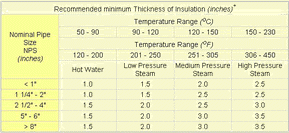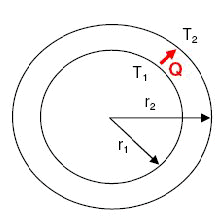Estimation of thickness for a pipe: Determine the minimum insulation thickness required for a pipe carrying steam at 180oC. The pipe size is 400 mm NB and the maximum allowable temperature of outer wall of insulation is 50oC. Thermal conductivity of the insulation material for the temperature range of the pipe can be taken as 0.04 W/m·K. The heat loss from steam per meter of pipe length has to be limited to 80 W/m.
For radial heat transfer by conduction across a cylindrical wall, the heat transfer rate is expressed by following equation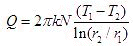T1 = 50oC
T2 = 180oC
r1 of 400 mm NB = 0.2032 m
k = 0.04 W/m·K
N = length of the cylinder
Q/N = Heat loss per unit length of pipe
Q/N = 80 W/m
Hence, inserting the given numbers in the radial heat transfer rate equation from above,
80 = 2pi × 0.04 × (180-50) ÷ ln(r2/0.2032)
ln(r2/0.2032) = 2pi × 0.04 × (180-50) / 80 = 0.4084
Hence, r2= r1 × e0.4084
r2= 0.2032 × 1.5044 = 0.3057 m
Hence, insulation thickness = r2 – r1
thickness = 305.7 – 203.2 = 102.5 mm

Some margin should be taken on the insulation thickness because if the conductive heat transfer rate happens to be higher than the convective heat transfer rate outside the insulation wall, the outer insulation wall temperature will shoot up to higher values than 500oC. Hence conductive heat transfer rate should be limited to lower values than estimates used in this sample problem. The purpose of this sample problem is to demonstrate radial heat conduction calculations and practical calculations of insulation thickness also require consideration of convective heat transfer on the outside of insulation wall.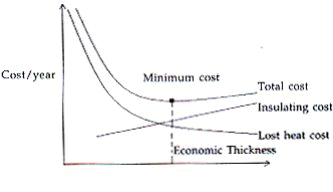Optimum thickness for a pipe: The economic thickness of insulation depends on the first cost (insulating cost) and maintenance cost of insulation and annual value of heat loss, which depends on the cost of producing the steam and thermal conductivity of the lagging. Generally thicker insulation will represent higher owing costs and lower heat loss costs.
Insulating Costs: The cost of insulation material per meter length is given as
= pi * [(R2)2 – (R1)2] *C1
Where C1 is the cost of lagging material in rupees per cubic meter.
Operating cost: The heat loss through insulation on the pipe per meter length is given by Q = 2*pi*k*[(T1-T2)/log (R2/R1)]
Where
T1 is inner surface temperature of insulation.
T2 is outer surface temperature of insulation.
R1 and R2 are inner and outer radii of insulation.
K is the thermal conductivity of insulating material.
This multiplied by cost of production of unit energy gives operating cost.
Optimum of thickness: When plotted it shows the lowest point, which gives the economic insulation thickness.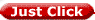to get all the information as a eBook